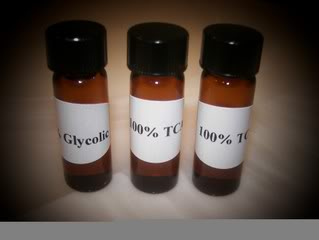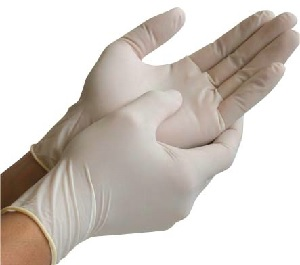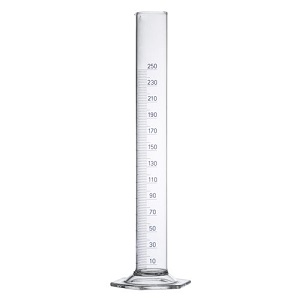# How to Dilute Lactic Acid,Glycolic Acid,Tca Peel,Salicylic Acid

#### EbonyNY

##### Moderator
Chemical Peels are a great way to get rid of hyperpigmentation and scars. There are many different chemicals and they penetrate the skin at different levels. Lactic acid, glycolic acid, tca peel and salicylic acid are mild chemical peels that can be done at home to improve the appearance of the skinAll chemical peels can be diluted with a simple chemistry formula C1 x V1=C2 x V2
You will need
• Measuring Cylinder (if you dont have a measurin cylinder you can use a syringe )
• Latex Gloves
• Water
• Beaker/Bowl or any small container
• Brush/StrawThe easiest way to dilute an acid solution without the calculation would be when you are trying to get half % of the solution. For example,let say you are trying to get a 40% solution out of an 80% solution.
1. Pour the solution into the measuring cylinder to get the volume you want . Let say 20ml, so you fill the cylinder up to 20ml with the acid solution
3.Rinse your cylinder,Now pour the water solution up to the volume you used for the acid (we used 20ml)
4.Pour the water into your container and mix it with the brush/straw
5. Now you have 40ml of 40% of the acid solution

To get other % other than the half(You would need the formula)
C1= Concentration of the acid you will be using
V1=Volume of the acid you will be using
C2=Concentration You want to get
V2=The volume you will get

First you would need to get the amount of water you would use so you should follow this steps and you would use the formula

For this example we would try to dilute a Lactic acid peel(Or any peel) of 100% to 30% .Let say it comes in a 40ml bottle and we are trying to dilute the whole thing or you can take out the amount you are trying to dilute by using the measuring cylinder
1. Multiply the original concentration of the acid and the amount you want to use = C1 x V1 (100% x 40ml)
2. Divide it by the concentration you are trying to get= C1 x V1/30%=(100% x 40ml) divided by 30%
3. That would be equal to 133.33ml approximatlet 133ml of water
4. So you would need to add 133ml to the 40ml bottle to get your 30% acid solution.

Last edited by a moderator:

#### bookWorM

##### New Member
Thank you for the formula

#### Femininity

##### Member
Just what i was looking for.

#### JayJay

##### Member
What peel are you gonna do ?

#### Femininity

##### Member
I'm doing a salicylic acid peel.

#### JayJay

##### Member
It so hard to find the equipments , where did you get yours ?

#### Femininity

##### Member
I got creative lol and i have some tubes and gloves at home.

#### JayJay

##### Member
They are really expensive online, i have to get creative too haha.

#### Femininity

##### Member
A simple ruler can do the job for measuring#### Lala18

##### Member
All this math and all this numbers lol, i will get it one day !!

#### sabah

##### New Member
Chemical Peels are a great way to get rid of hyperpigmentation and scars. There are many different chemicals and they penetrate the skin at different levels. Lactic acid, glycolic acid, tca peel and salicylic acid are mild chemical peels that can be done at home to improve the appearance of the skin

View attachment 34
All chemical peels can be diluted with a simple chemistry formula C1 x V1=C2 x V2
You will need
• Measuring Cylinder (if you dont have a measurin cylinder you can use a syringe )
• Latex Gloves
• Water
• Beaker/Bowl or any small container
• Brush/Straw
View attachment 35View attachment 36

The easiest way to dilute an acid solution without the calculation would be when you are trying to get half % of the solution. For example,let say you are trying to get a 40% solution out of an 80% solution.
1. Pour the solution into the measuring cylinder to get the volume you want . Let say 20ml, so you fill the cylinder up to 20ml with the acid solution
3.Rinse your cylinder,Now pour the water solution up to the volume you used for the acid (we used 20ml)
4.Pour the water into your container and mix it with the brush/straw
5. Now you have 40ml of 40% of the acid solution

To get other % other than the half(You would need the formula)
C1= Concentration of the acid you will be using
V1=Volume of the acid you will be using
C2=Concentration You want to get
V2=The volume you will get

First you would need to get the amount of water you would use so you should follow this steps and you would use the formula

For this example we would try to dilute a Lactic acid peel(Or any peel) of 100% to 30% .Let say it comes in a 40ml bottle and we are trying to dilute the whole thing or you can take out the amount you are trying to dilute by using the measuring cylinder
1. Multiply the original concentration of the acid and the amount you want to use = C1 x V1 (100% x 40ml)
2. Divide it by the concentration you are trying to get= C1 x V1/30%=(100% x 40ml) divided by 30%
3. That would be equal to 133.33ml approximatlet 133ml of water
4. So you would need to add 133ml to the 40ml bottle to get your 30% acid solution.
this is the easiest way to dilute !!!! I have been looking for a long time.

#### Lloyd Engineer

##### New Member
Chemical Peels are a great way to get rid of hyperpigmentation and scars. There are many different chemicals and they penetrate the skin at different levels. Lactic acid, glycolic acid, tca peel and salicylic acid are mild chemical peels that can be done at home to improve the appearance of the skin

View attachment 34
All chemical peels can be diluted with a simple chemistry formula C1 x V1=C2 x V2
You will need
• Measuring Cylinder (if you dont have a measurin cylinder you can use a syringe )
• Latex Gloves
• Water
• Beaker/Bowl or any small container
• Brush/Straw
View attachment 35 View attachment 36

The easiest way to dilute an acid solution without the calculation would be when you are trying to get half % of the solution. For example,let say you are trying to get a 40% solution out of an 80% solution.
1. Pour the solution into the measuring cylinder to get the volume you want . Let say 20ml, so you fill the cylinder up to 20ml with the acid solution
3.Rinse your cylinder,Now pour the water solution up to the volume you used for the acid (we used 20ml)
4.Pour the water into your container and mix it with the brush/straw
5. Now you have 40ml of 40% of the acid solution

To get other % other than the half(You would need the formula)
C1= Concentration of the acid you will be using
V1=Volume of the acid you will be using
C2=Concentration You want to get
V2=The volume you will get

First you would need to get the amount of water you would use so you should follow this steps and you would use the formula

For this example we would try to dilute a Lactic acid peel(Or any peel) of 100% to 30% .Let say it comes in a 40ml bottle and we are trying to dilute the whole thing or you can take out the amount you are trying to dilute by using the measuring cylinder
1. Multiply the original concentration of the acid and the amount you want to use = C1 x V1 (100% x 40ml)
2. Divide it by the concentration you are trying to get= C1 x V1/30%=(100% x 40ml) divided by 30%
3. That would be equal to 133.33ml approximatlet 133ml of water
4. So you would need to add 133ml to the 40ml bottle to get your 30% acid solution.
Had to register because this is wrong and couldn't leave it, especially as it's a front page search engine result.... Close and still a handy equation though, just the math is off.
This :
"So you would need to add 133ml to the 40ml bottle to get your 30% acid solution."
No that's incorrect, but on the safer side at least...

Correct calculation =

100% to 30% comes as 40ml

C1 x V1=C2 x V2
100% x 40ml = 30% x v2
4000 = 4000/30 = 133.33ml

V2 = 133.33 ml TOTAL fluid.
133.33ml - 40ml = 93.33 ml
To get total of 133ml dilute at 30% concentration.

It's math, make sure your equation is balanced with a check at the end, if it isn't, you made a mistake

Source = Degree in Engineering

#### Lloyd Engineer

##### New Member
Balance equation check =

C1 x V1=C2 x V2

100% x 40ml = 30% x 133ml
4000 = 4000 Correct.

#### deeanna01

##### New Member
Hey Lloyd, From one math idiot to an obvious genius, can you tell me how to dilute 99% tca powder to 25%.
How much water if I am making big batch with 1 kg powder?